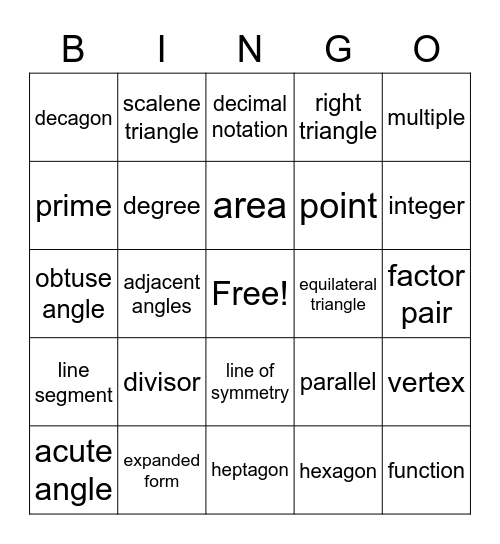# Math PSSA PrepThis bingo card has a free space and 29 words: acute angle, acute triangle, adjacent angles, composite number, decagon, decimal notation, degree, divisor, equilateral triangle, expanded form, factor pair, function, heptagon, hexagon, integer, isoceles triangle, line of symmetry, line segment, multiple, obtuse angle, parallel, perpendicular, point, prime, right triangle, scalene triangle, vertex, area and denominator.

## Play Online